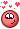## Recommended Posts

Posted (edited)

Hi,

I am trying to make a cellular automata sim a la John Conway. The vex version works fine and I think it is true to the original; it runs the same each time for a given set of initial conditions, as you would expect. However, when I try to use the code in openCL there is some variation, from the first frame or whenever I 'Reset Simulation'. I can't work out where this variation comes from and why the algorithm doesn't run as you would expect.

I have tried using int, and float for the parameters with 32 bit and 64 bit precision, this doesn't fix the problem. Is there some kind of rounding which happens in Open CL but not vex? Ideally I would like the VEX and Open CL versions to be the same - is that possible?

Please see attached hip. Thanks so much.

Edited by jimmojones

##### Share on other sites
Posted (edited)

Try thishttps://zhuanlan.zhihu.com/p/54396666
https://zhuanlan.zhihu.com/p/79503871

Edited by Librarian
Fun

##### Share on other sites

your opencl code does not work in you scene, i didn;t have to figure more...
btw. more i've found even openCL differences between houdini17 and 18.

i hope sidefx will give a cuda wrangle node in futures releases. (openCL is pretty much abandon )

##### Share on other sites

Thanks, Tesan and Heribert.

Although my question is really why doesn't the OpenCL implementation give the same results as VEX given the same initial conditions? And why would the openCL vary even when just pressing "Reset Simulation", as all the parameters are the same?

I have tried using different combinations of int and float, but the rules should result in 1 or 0 given the number of neighbours. I can't see where the error would creep in.

Please see attached file, it should work, I am using Houdini 18.0.287. The openCL code is below:

```float convolve(int idx, int resx, int resy, global float* a)
{
// Mirror coords.
int l = 1;
int r = 1;
if (idx % resx == 0)
l = -l;
else if (idx % resx == resx - 1)
r = -r;
int b = resx;
int t = resx;
if (idx < resx)
b = -b;
else if (idx >= resx * (resy - 1))
t = -t;

// Return weighted sum.
return a[idx+t-l] * 2 +
a[idx+t]   * 2 +
a[idx+t+r] * 2 +
a[idx-l]   * 2 +
a[idx]     * 1 +
a[idx+r]   * 2 +
a[idx-b-l] * 2 +
a[idx-b]   * 2 +
a[idx-b+r] * 2;
}

kernel void gol(
int ca_length,
global float * ca ,
int resx_length,
global int * resx ,
int resy_length,
global int * resy
){
int idx = get_global_id(0);
if (idx >= ca_length)
return;
int array[] = {0,0, // number of neighbours = 0
0,0, // number of neighbours = 1
0,1, // number of neighbours = 2
1,1, // number of neighbours = 3
0,0,
0,0,
0,0,
0,0,
0,0};
float neighbours = convolve(idx, resx[idx], resy[idx], ca);
int rule = int(neighbours);
ca[idx] = array[rule];

}```

GOL_openCL_vex_02.hipnc

##### Share on other sites

The OpenCL node errors in your scene for some reason (im on 18.0.416), so can't test. But your problem is most likely reading and writing to the same attribute in the same kernel. You want to use a Write Back Kernel for this. If you hover over the "Use Write Back Kernel" it explains a bit what happens.

##### Share on other sites

Thanks, David!!!

Adding a Write Back Kernel fixed the problem - the simulation runs the same each time and is identical to VEX.  Interestingly, the OpenCL solver runs about 50% slower than the VEX on 1 million points..

## Create an account

Register a new account